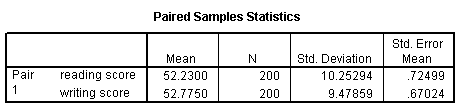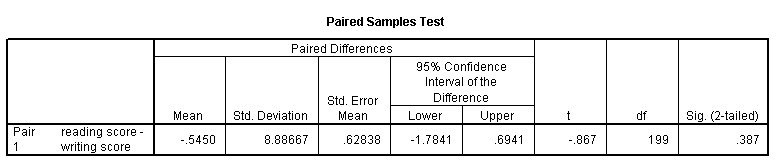## Paired t-test

A paired (samples) t-test is used when you have two related observations (i.e., two observations per subject) and you want to see if the means on these two normally distributed interval variables differ from one another. For example, using the hsb2 data file we will test whether the mean of read is equal to the mean of write.

t-test pairs = read with write (paired).These results indicate that the mean of read is not statistically significantly different from the mean of write (t = -0.867, p = 0.387).